# KVPY-SX 2016 Physics Paper with Solutions

The first step towards the KVPY Exam Preparation is to gather and solve the previous year question papers. Here, students will get access to the KVPY 2016 question papers along with solutions for each question. Solving the previous years’ papers will give students a much better idea about the pattern, difficulty level, marking scheme of the questions. In addition, the answers have been prepared by our subject experts making it easy for students to understand the topics more easily. Students will also get to know how much preparation time will be required before writing the exam. Students can easily download the solutions in PDF format from the bottom of the page.

### KVPY SX 2016 - Physics

Question 1. Physical processes are sometimes described visually by lines. Only the following can cross -

1. a) Streamlines in fluid flow
2. b) Lines of forces in electrostatics
3. c) Rays in geometrical optics
4. d) Lines of force in magnetism

Solution:

[a] Streamlines are always parallel to each other.

[b] If line of forces in electrostatics crosses each other then, at the point of intersection there will be two direction of electric field intensity which is not possible.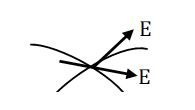[c] In case of reflection or refraction of light rays, the image formation is the meeting/crossing of light ray’s through a point.

[d] If lines of force in magnetism cross each other then, at the point of intersection there will be two direction of magnetic field intensity which is not possible.

Question 2. A uniform ring of radius R is moving on a horizontal surface with speed v and then climbs up a ramp of inclination 30° to a height h. There is no slipping in the entire motion. Then h is

1. a) v2/2g
2. b) v2/g
3. c) 3v2/2g
4. d) 2v2/g

Solution:

As there is no slipping, energy will be conserved

∴ Initial kinetic energy = final potential energy

½ Iω2 + ½ mv2 = mgh ….(1)

m = mass, h = height, I = moment or inertia = mR2, ω(angular velocity) = v / R

∴ From equation (1)

$\frac{1}{2}(mR^{2})\left ( \frac{v^{2}}{R^{2}} \right )+\frac{1}{2}mv^{2} = mgh$

$\frac{1}{2}mv^{2}+\frac{1}{2}mv^{2} = mgh$

$h = \frac{v^{2}}{g}$

Question 3. A gas at initial temperature T undergoes sudden expansion from volume V to 2V. Then -

1. a) The process is adiabatic
2. b) The process is isothermal
3. c) The work done in this process is nRTλne(2) where n is the number of moles of the gas.
4. d) The entropy in the process does not change

Solution:

Whenever there is a sudden expansion or abrupt or rapid change of volume in a process then the process will be adiabatic, since there is no heat change.

For example: bursting of a tire.

For adiabatic process: TVγ= constant; where γ = CP / CV

From first law of thermodynamics dQ = dU+ dW

∵ dQ = 0

∴ dU = dW or –dU = dW (+ve because of expansion)

dU = –ve so dT = –ve ; dU = change in internal energy

i.e. Temperature will decrease so process is not isothermal

The given option (c) is applicable for isothermal process because for isothermal process work done Wisothermal = nRT loge VF / Vi so, it is incorrect.

Now, as entropy for system is given by

dS = dQ / dT

Substituting the value of dQ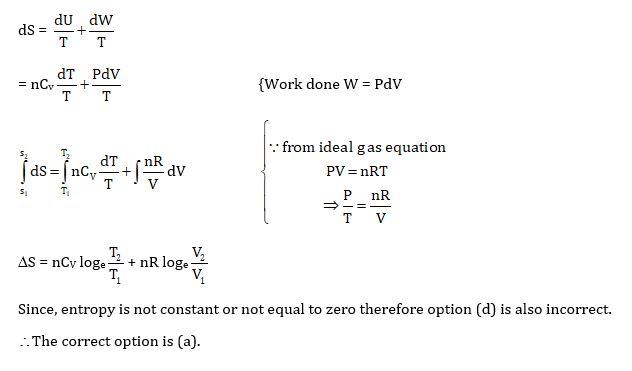Question 4. Photons of wavelength λ are incident on a metal. The most energetic electrons ejected from the metal are bent into a circular arc of radius R by a perpendicular magnetic field having a magnitude B. The work function of the metal is (Where symbols have their usual meanings) –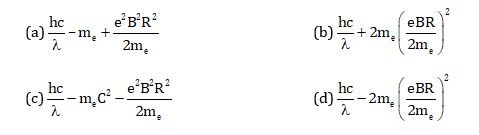Solution:

Let us suppose, the energy of the photon is hv, λ is work function of the metal, (KE)max is the maximum kinetic energy of the ejected electron.

Now from Einstein photo electric equation,

hv – ϕ = (K.E.)max.

⇒ ϕ = – (K.E.)max. ….(1) { ∵ v = c / λ }

Given that electron bent into a circular arc of radius R by a perpendicular magnetic field having a magnitude B, then it will experience a force perpendicular to the direction of velocity of electron and it is equal to centripetal force.

So, $qVB = \frac{m_{e}v^{2}}{R}$, me = mass of the electron, q = charge of the electron = e

$v = \frac{eBR}{m_{e}}$

∵ K.E. = ½ mv2

Substituting the value of v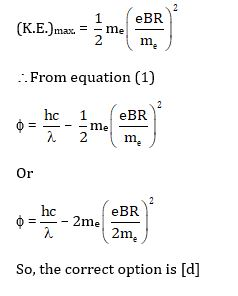Question 5. A container is divided into two equal part I and II by a partition with a small hole of diameter d. The two partitions are filled with same ideal gas, but held at temperatures TI = 150 K and TII = 300 K by connecting to heat reservoirs. Let λI and λII be the mean free paths of the gas particles in the two parts such that d >> λ I and d >> λII. Then λ III is close to -

1. a) 0.25
2. b) 0.5
3. c) 0.7
4. d) 1.0

Solution:

As partition has hole of diameter d, mean pressure between both sections is equal.

∵ Mean force path (λ) is given by = $\frac{1}{\sqrt{2\pi r^{2}n_{v}}}$

r = radius of the gas particle

nV = number density of gas i.e. = $\frac{N}{V} = \frac{(Total\: no\: of\: particle)}{(Volume)}$

∵ From ideal gas equation PV = nRT = N / NA RT = NKB T

NA = Avogadro number; KB = Boltzmann constant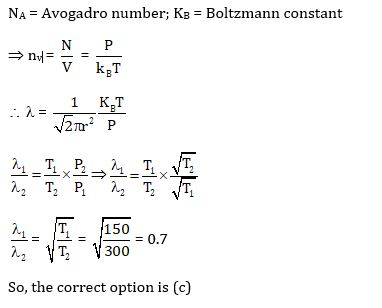Question 6. A conducting bar of mass m and length ℓ moves on two frictionless parallel rails in the presence of a constant uniform magnetic field of magnitude B directed into the page as shown in the figure. The bar is given an initial velocity v0 towards the right at t = 0. Then the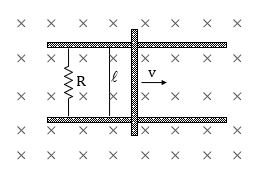1. a) Induced current in the circuit is in the clockwise direction
2. b) Velocity of the bar decreases linearly with time
3. c) Distance the bar travels before it comes to a complete stop is proportional to R
4. d) Power generated across the resistance is proportional to ℓ

Solution:

Given mass of the bar = m, length of the conducting bar = ℓ, the magnitude of magnetic field = B

When bar moves on frictionless parallel rails then area of left side will increase therefore, flux will also increase.

Since, magnetic field in inward the plane so according to Lenz law it will try to reduce the magnetic flux and magnetic field will be outside the plane so current will be in anti-clockwise direction.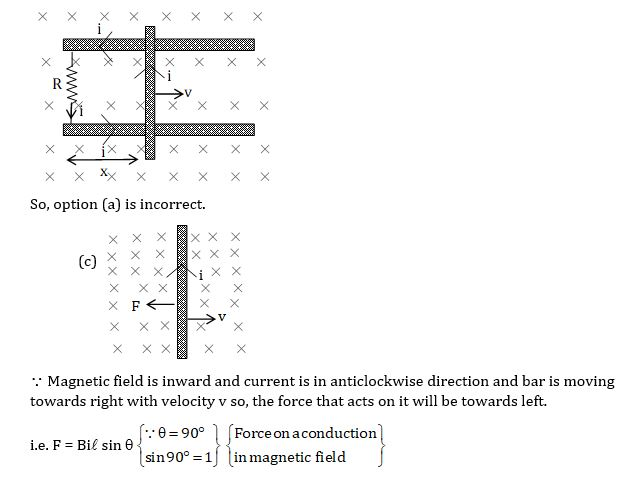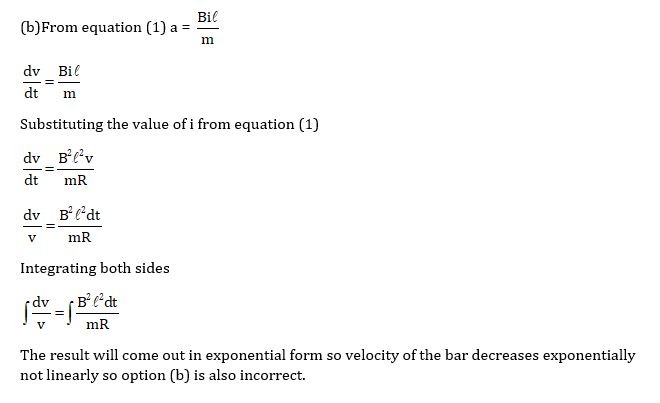F = Biℓ

So, acceleration will be

a = F/ m = Bi / m ……(1)

∵ flux Φ = B. A.

∴ Φ = B x

∴ differentiating with respect to td) ∵ Power P = i2R

Substituting the value of i

$P = \frac{B^{2}l^{2}v^{2}}{R^{2}}.R$

$P = \frac{B^{2}l^{2}v^{2}}{R}$

P α2

So, option (d) is incorrect.

Question 7. A particle with total mechanical energy, which is small and negative, is under the influence of a one-dimensional potential U(x) = x4/4 – x2/2 J, where x is in meters. At time t = 0s, it is at x = –0.5 m. Then at a later time it can be found

1. a) Anywhere on the x axis
2. b) Between x = – 1.0 m to x = 1.0 m
3. c) Between x = – 1.0 m to x = 0.0 m
4. d) Between x = 0.0 m to x = 1.0 m

Solution:

Given, one dimensional potential of particle U(x) = $\frac{x^{4}}{4}=\frac{x^{2}}{2}J$ …..(1)

At t = 0, x = –0.5

Substituting the value of t and x in equation (1)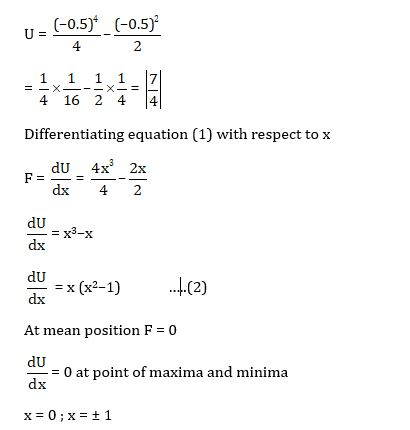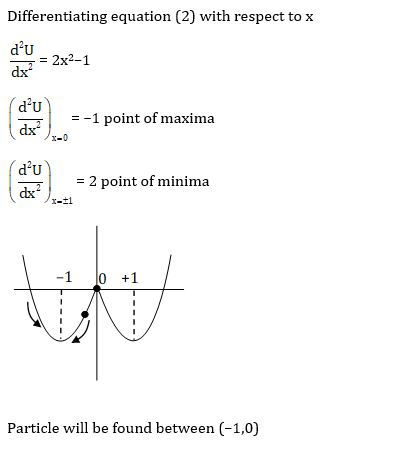Question 8. A nurse measures the blood pressure of a seated patient to be 190 mm of Hg -

1. a) The blood pressure at the patient's feet is less than 190 mm of Hg
2. b) The actual pressure is about 0.25 times the atmospheric pressure
3. c) The blood pressure at the patient's neck is more than 190 mm of Hg
4. d) The actual pressure is about 1.25 times the atmospheric pressure

Solution:

∵Blood pressure is gauge pressure = 190 mm Hg

We know atmospheric pressure = 760 mm Hg.

So, actual pressure will be = gauge pressure + atmospheric pressure

= 190 + 760

= 950 mm Hg

Now $\frac{P_{actual}}{P_{atm}} = \frac{950}{760} = 1.25$

⇒ Pactual = 1.25 Patm

So, the actual pressure is about 1.25 times the atmospheric pressure.

Question 9. A particle at a distance of 1 m from the origin starts moving such that dr/d θ = r, where (r, θ) are polar coordinates. Then the angle between resultant velocity and tangential velocity component is

1. a) 30 degrees
2. b) 45 degrees
3. c) 60 degrees
4. d) Dependent on where the particle is

Solution:

Velocity is given by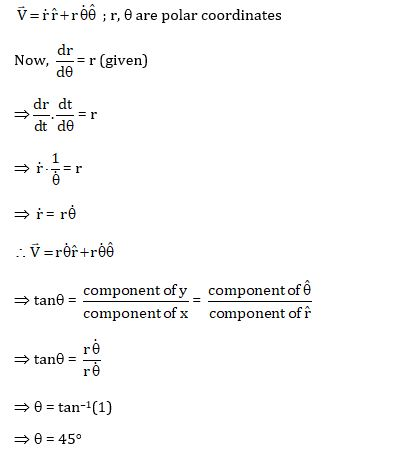Question 10. Electrons accelerated from rest by an electrostatic potential are collimated and sent through a Young's double slit setup. The fringe width is w. If the accelerating potential is doubled then the width is now close to

1. a) 0.5 w
2. b) 0.7 w
3. c) 1.0 w
4. d) 2.0 w

Solution:

In Young’s double slit experiment fringe width is given by $\beta = \frac{\lambda D}{d}\Rightarrow \beta \alpha \lambda$ ….(1)

Where λ = wavelength of the particle (electron)

D = Distance between the slit and screen

d = distance between the slits

Let, initial potential = ∆Vi

Final potential = ∆Vf

So, according to the question ∆Vf = 2 ∆Vi

From de Broglie’s wavelength $\lambda = \frac{h}{p}= \frac{h}{mv} = \frac{h}{\sqrt{qm\Delta V}}$

$\Rightarrow \lambda\: \alpha \frac{1}{\Delta V}$

⇒ from equation (1) $\beta \: \alpha \frac{1}{\Delta V}$

So βf =√∆Vf , βi = √∆Vi

∵ βi = w (given)

∴ Substituting the values of βi, ∆Vi and ∆Vf, we get

βf = w. 1/√2

βf = 0.7 w

Question 11. A metallic sphere is kept in between two oppositely charged plates. The most appropriate representation of the field lines is –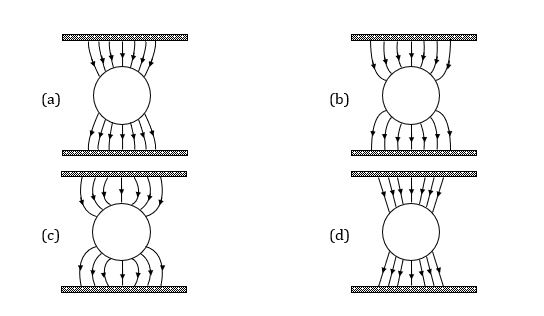Solution:

Electric field lines should be perpendicular to surface of metal so the correct option is [b].

Question 12. An electron with kinetic energy E collides with a hydrogen atom in the ground state. The collision will be elastic

1. a) For all values of E
2. b) For E < 10.2 eV
3. c) For 10.2 eV < E < 13.6 eV only
4. d) For 0 < E < 3.4 eV only

Solution:

The hydrogen atom in ground state will only absorb energy greater than 10.2 eV. When electron with kinetic energy, EeV collides with hydrogen atom in ground state, if energy of an electron is absorbed then collision will be inelastic. If there is no absorption of energy (i.e. E < 10.2 eV) then collision will be elastic.

Question 13. The continuous part of X-ray spectrum is a result of the

1. a) Photoelectric effect
2. b)Raman effect
3. c) Compton effect
4. d) Inverse photoelectric effect

Solution:

The process of x-ray emission by incoming electron is also known as inverse photoelectric effect. The continuous part of x-ray spectrum is result of the inverse photo electric effect.

Question 14. Thermal expansion of a solid is due to the

1. a) Symmetric characteristic of the inter atomic potential energy curve of the solid.
2. b) Asymmetric characteristic of the inter atomic potential energy curve of the solid.
3. c) Double well nature of the inter-atomic potential energy curve of the solid.
4. d) Rotational motion of the atoms of the solid.

Solution:

Thermal expansion is a tendency of matter to change its shape, area and volume in response to change in temperature. Temperature is a monotonic function of average kinetic energy of a substance. When a substance is heated, the kinetic energy of its molecules increases. Thus, the molecules begin vibrating/moving more and usually maintain a greater average separation. Asymmetric characteristic of the inter atomic potential energy curve of a solid is the reason for thermal expansion.

Question 15. An electron and a photon have same wavelength of 10–9 m. If E is the energy of the photon and p is the momentum of the electron, the magnitude of E/p in SI units is

1. a) 1.00 × 10–9
2. b) 1.50 × 108
3. c) 3.00 × 108
4. d) 1.20 × 107

Solution:

Given,

Wavelength of photon and electron are equal

λp = λe = 10–9m

E = energy of photon

P = momentum of electron

From plank’s equation

(Energy) E = hc / λp ⇒ λp = hc / E; h = plank’s constant, c = speed of light (3×108 m/s)

From de-Broglie’s equation,

λe = h/p

Since, λp = λe

⇒ hc/E = h/p

⇒ E / p= c = 3 × 108 m/s

Question 16. If one takes into account finite mass of the proton, the correction to the binding energy of the hydrogen atom is approximately (mass of proton = 1.60 × 10–27 kg, mass of electron = 9.10 × 10–31 kg)-

1. a) 0.06%
2. b) 0.0006 %
3. c) 0.02 %
4. d) 0.00 %

Solution: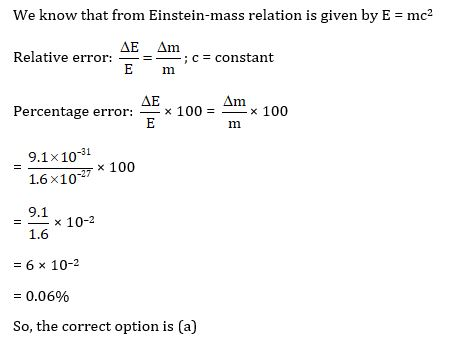Question 17. A monochromatic light source S of wavelength 440 nm is placed slightly above a plane mirror M as shown. Image of S in M can be used as a virtual source to produce interference fringes on the screen. The distance of source S from O is 20.0 cm, and the distance of screen from O is 100.0 cm (figure is not to scale). If the angle  = 0.50 × 10–3 radians, the width of the interference fringes observed on the screen is;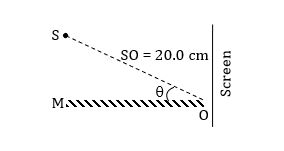1. a) 2.20 mm
2. b) 2.64 mm
3. c) 1.10 mm
4. d) 0.55 mm

Solution:

Given wavelength, λ = 440 nm = 440 × 10–9 m = 440 × 10–7cm

SO = 20 cm = 20 × 10–2m

θ = 0.5 × 10–2 radians (very small)

To find width of fringes

S and SI are source of Young’s double slit experiment (YDSE)

D = SO cos θ + 100 = 20 × 1 + 100 = 120cm

d = 2 × SO sin θ = 2 × 20 × 0.5 × 10–3 = 2× 10–2 cm

Fringe width

β = λ d / D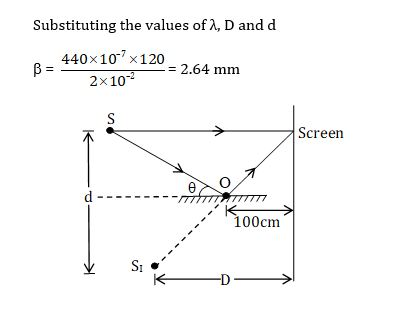Question 18. A nuclear fuel rod generates energy at a rate of 5 × 108 Watt/m3. It is in the shape of a cylinder of radius 4.0 mm and length 0.20 m. A coolant of specific heat 4 × 103 J/(kg-K) flows past it at a rate of 0.2 kg/s. The temperature rise in this coolant is approximately -

1. a) 2°C
2. b) 6°C
3. c) 12°C
4. d) 30°C

Solution:

Given: Rod generates energy at a rate = 5 × 108Watt/m3

Shape is cylindrical, radius = 4mm

Length = 0.20 m

Specific heat of coolant(s) = 4 × 103 $\frac{J}{kg - K}$

Rate of coolant $\left (\frac{dm}{dt} \right )$ = 0.2 kg/s

Temperature rise of coolant (∆T) = ?

∵We know that heat is given by

Q = ms ∆T

So $\frac{dq}{dt} = \frac{dm}{dt}S\Delta T$ …..(1)

$\frac{dq}{dt}$ = 5 × 108 × volume of rod

= 5 × 108 × π r2

= 5 × 108 × π × (4)2 × 10–6 × .2

= 5 × 10 × π × 16 × 2 = 1600 π

Substituting the values in equation (1),

0.2 × 4 × 10∆T = 1600π

8× 102∆T = 16× 102 π

∆T = 3.14 × 2

∆T = 6.28°C

So, the correct option is (b).

Question 19. A hearing test is conducted on an aged person. It is found that her threshold of hearing is 20 decibels at 1 kHz and it rises linearly with frequency to 60 decibels at 9 kHz. The minimum intensity of sound that the person can hear at 5 kHz is-

1. a) 10 times than that at 1 kHz
2. b) 100 times than that at 1 kHz
3. c) 0.5 times than that at 9 kHz
4. d) 0.05 times than that at 9 kHz

Solution:

Loudness of sound is measured as β = 10 log10 $\left ( \frac{I}{I_{o}} \right )$ ….. (1)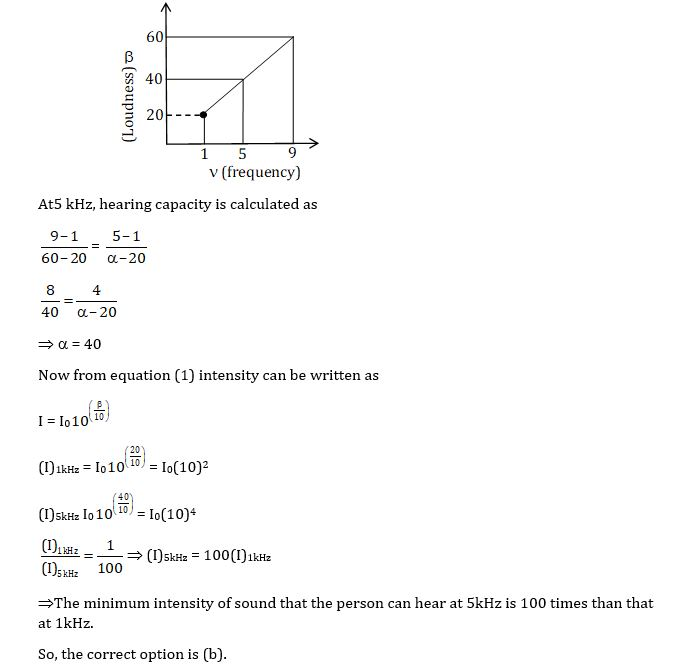Question 20. Two infinitely long parallel wires carry currents of magnitude I1 and I2 and are at a distance 4 cm apart. The magnitude of the net magnetic field is found to reach a non-zero minimum value between the two wires and 1 cm away from the first wire. The ratio of the two currents and their mutual direction is

1. a) $\frac{I_{2}}{I_{1}}$ = 9, antiparallel
2. b) $\frac{I_{2}}{I_{1}}$ = 9, parallel
3. c) $\frac{I_{2}}{I_{1}}$ = 3, antiparallel
4. d) $\frac{I_{2}}{I_{1}}$ = 3, parallel

Solution:

Given, two wires are infinity long.

Current in wires are I1 and I2 respectively.

Distance between wires = 4cm

Case-(I): When current in both the wires is in same direction.

Case-(II): When current in both the wires is in opposite direction.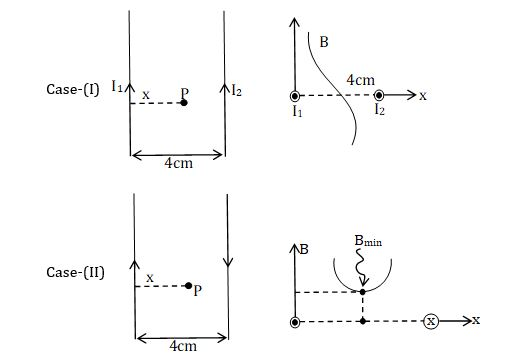In both the cases, when we draw graph we see only in case-II we get the minimum value of B.

So, in case II →

Net magnetic field at point P

Bp = magnetic field due to wire 1 at P + magnetic field due to wire 2 at P.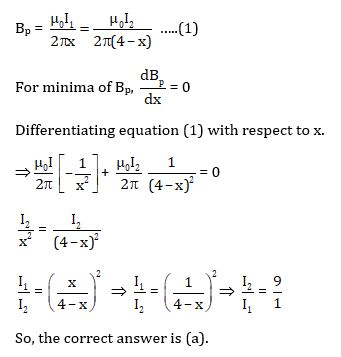Question 21. A light balloon filled with helium of density ρHe is tied to a long light string of length l and the string is attached to the ground. If the balloon is displaced slightly in the horizontal direction from the equilibrium and released then.

1. a) The balloon undergoes simple harmonic motion with period $2\pi \sqrt{\left ( \frac{\rho_{air}}{\rho_{air} - \rho_{He}} \right )\frac{l}{g}}$
2. b) The balloon undergoes simple harmonic motion with period $2\pi \sqrt{\left ( \frac{\rho_{air}- \rho_{He}}{\rho_{air}} \right )\frac{l}{g}}$
3. c) The balloon undergoes simple harmonic motion with period $2\pi \sqrt{\left ( \frac{\rho_{He}}{\rho_{air} - \rho_{H_{e}}} \right )\frac{l}{g}}$
4. d) The balloon undergoes conical oscillations with period $2\pi \sqrt{\left ( \frac{\rho_{air} + \rho_{He}}{\rho_{air} - \rho_{H_{e}}} \right )\frac{l}{g}}$

Solution: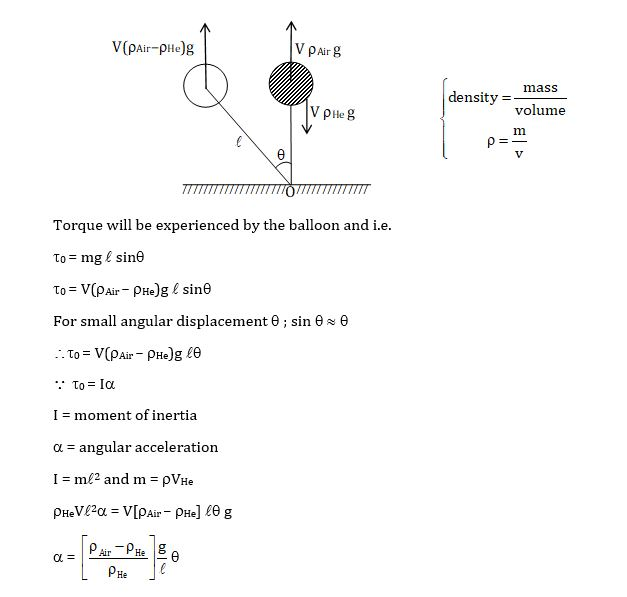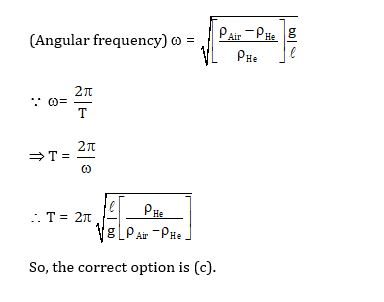Question 22. Consider a cube of uniform charge density ρ. The ratio of electrostatic potential at the center of the cube to that at one of the corners of the cube is

1. a) 2:1
2. b) √3/2:3
3. c) √2:2
4. d) 1:1

Solution:

Given: Charge density of cube = ρ

Let, side of the cube = a

∴ Potential is given by (Let, at the center of cube potential is V).

So, V =kq / r ⇒ α q/r; q = charge, r = distance

Here, r = a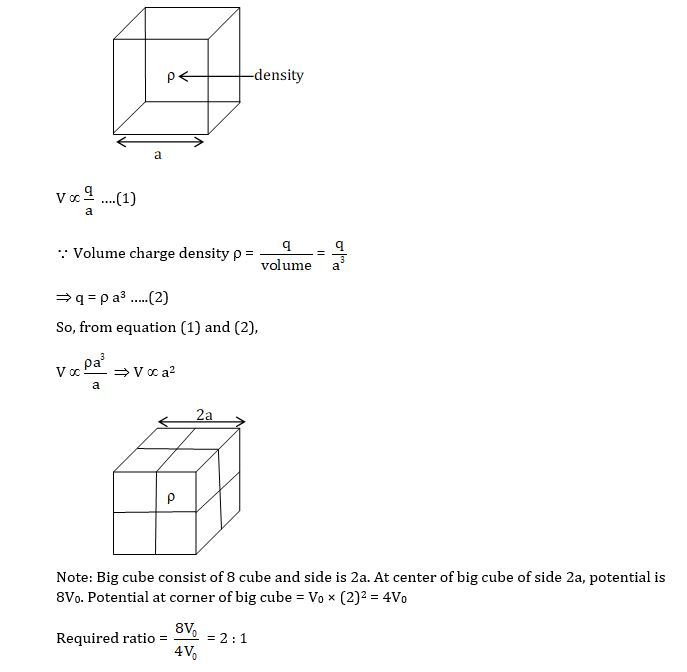Question 23. Two infinitely long wires each carrying current I along the same direction is made into the geometry as shown in the figure. The magnetic field at the point P is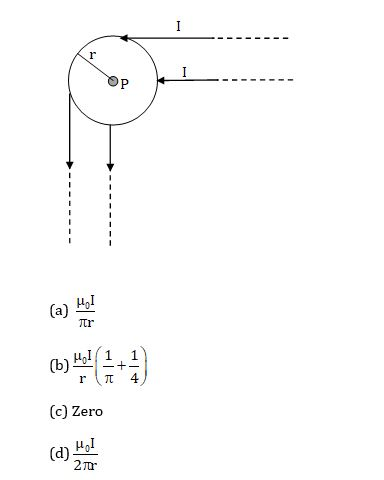Solution: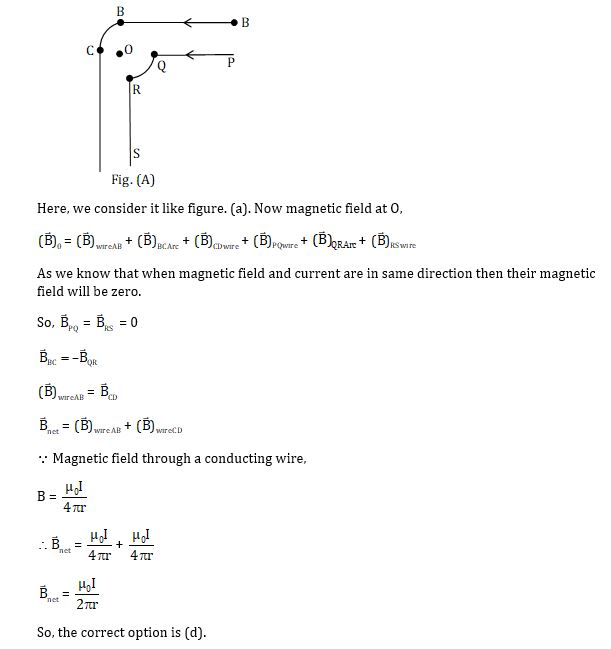Question 24. A photon of wavelength λ is absorbed by an electron confined to a box of length √(35hλ/8mc).As a result, the electron makes a transition from state k = 1 to the state n. Subsequently the electron transits from the state n to the state m by emitting a photon of wavelength λ' = 1.85 λ. Then

1. a) n = 4; m = 2
2. b) n = 5; m = 3
3. c) n = 6; m = 4
4. d) n = 3; m = 1

Solution: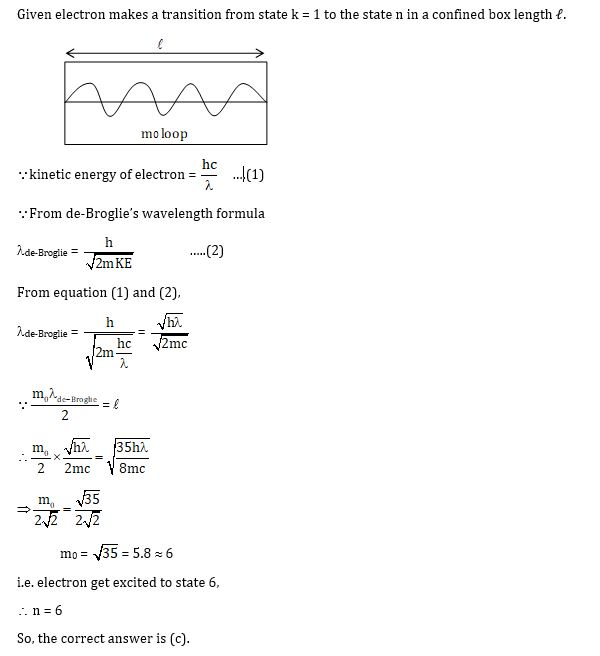Question 25. Consider two masses with m1> m2 connected by a light inextensible string that passes over a pulley of radius R and moment of inertia I about its axis of rotation. The string does not slip on the pulley and the pulley turns without friction. The two masses are released from rest separated by a vertical distance 2h. When the two masses pass each other, the speed of the masses is proportional to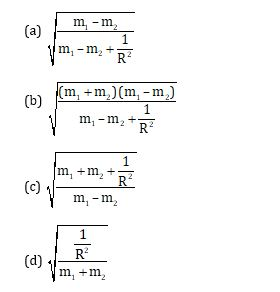Solution:

System will be = Pulley + Earth

Total energy is conserved so it implies that

Gain in kinetic energy + loss in potential energy.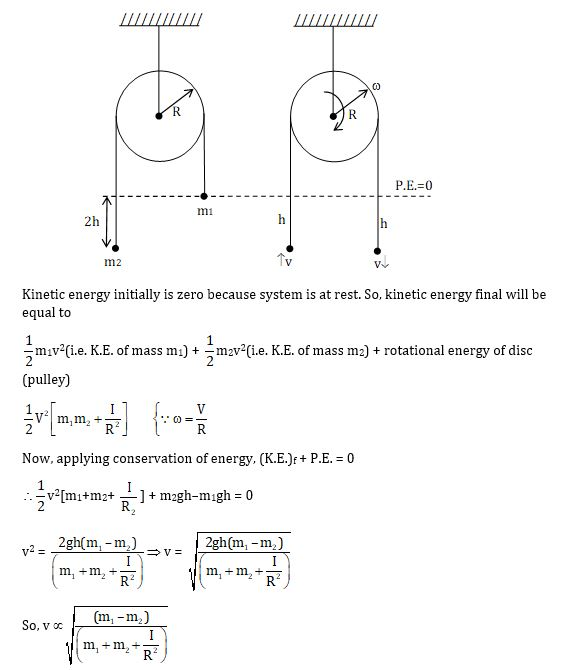Question 26. An ideal gas is taken reversibly around the cycle a-b-c-d-a as shown on the T (temperature) – S (entropy) diagram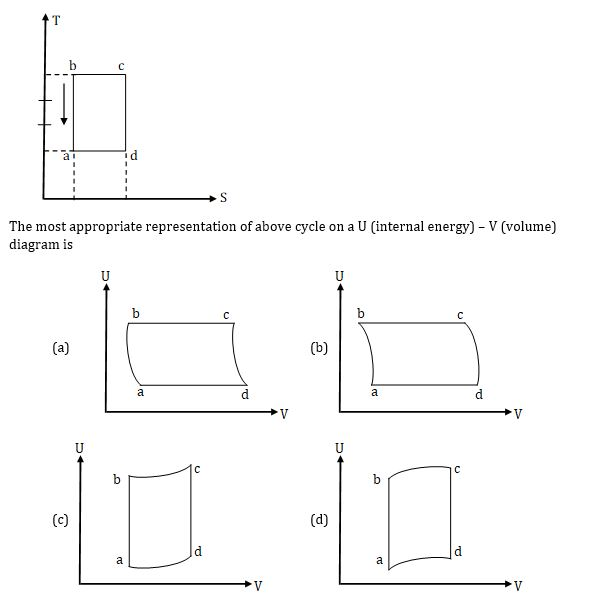Solution:

Given, curve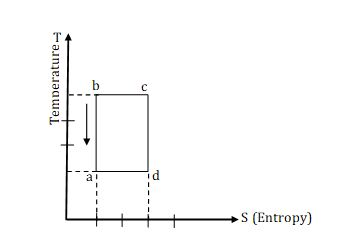As we can see from diagram that bc process is an isothermal process i.e. temperature remains constant at bc.

∵ Change in internal energy also remains constant.

cd process is an isentropic process so S(entropy) remains constant.

An isentropic process is a thermodynamic process, in which the entropy of the fluid or gas remains constant. It means the isentropic process is a special case of an adiabatic process in which there is no transfer of heat or matter. It is a reversible adiabatic process.

For a → b, temperature will be increased

For c → d, temperature will be decreased

So, the graph will be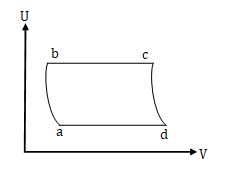Therefore, the correct option is (a).

Question 27. The heat capacity of one mole of an ideal is found to be CV = 3R (1 + aRT)/2 where a is a constant. The equation obeyed by this gas during a reversible adiabatic expansion is -

1. a) TV3/2 eaRT = constant
2. b) TV3/2 e3aRT/2 =constant
3. c) TV3/2= constant
4. d) TV3/2 e2aRT/3= constant

Solution:

Given, heat capacity of one mole of an ideal gas (Cv) = $\frac{3R\left ( 1+aRT \right )}{2}$

In reversible adiabatic process, the equation is given by

TVγ–1 = C (constant) ……(1)

Where T = temperature, V = volume

γ= ration of specific heats i.e. γ = Cp / Cv

The relation between γ and degree of freedom (f) is given by γ = 1+ 2/f …..(2)

Now from equation (1) and (2),

$TV^{1+\frac{2}{f}-1} = C$

$TV^{\frac{2}{f}} = C$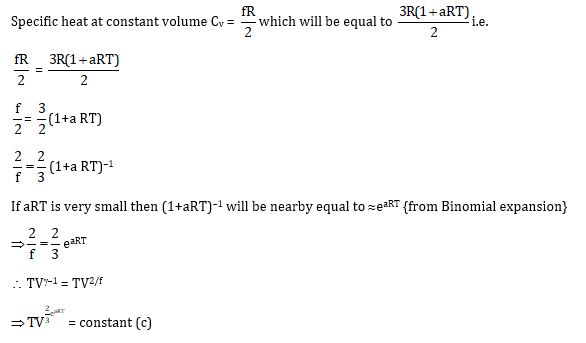Question 28. If the input voltage Vi to the circuit below is given by Vi(t) = A cos(2πft), the output voltage is given by Vo(t) = B cos(2πft + ϕ) –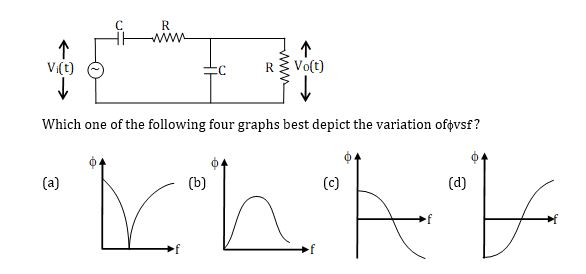Solution: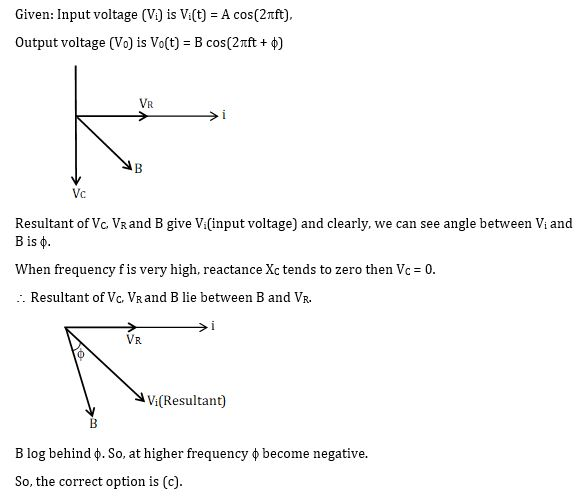Question 29. A glass prism has a right-triangular cross section ABC, with ∠A = 90º. A ray of light parallel to the hypotenuse BC and incident on the side AB emerges grazing the side AC. Another ray, again parallel to the hypotenuse BC, incident on the side AC suffers total internal reflection at the side AB. Which one of the following must be true about the refractive index µ of the material of the prism?

1. a) $\sqrt{\frac{3}{2}}< \mu < \sqrt{2}$
2. b) $\mu > \sqrt{3}$
3. c) $\mu < \sqrt{\frac{3}{2}}$
4. d) $\sqrt{2}< \mu < \sqrt{3}$

Solution: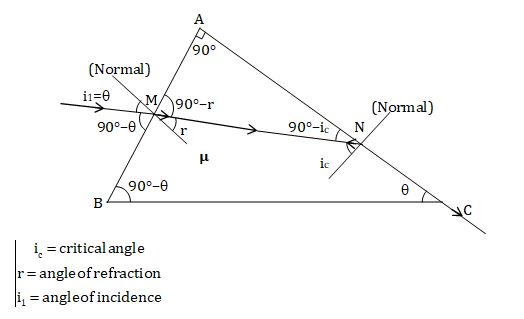At M, $\frac{sini_{1}}{sinr} = \mu$ (from Snell’s law)

In this case, from triangle AMN

90° + 90° – r + 90–ic = 180°

r + ic = 90°

r = 90°–ic

From Snell’s law, $\frac{sini_{1}}{sinr} = \mu$

$\frac{sin\theta }{sin(90-i_{c})} = \mu$

$\frac{sin\theta }{cosi_{c}} = \mu$

$\frac{sin\theta }{\sqrt{1 - sin^{2}i_{c}}} = \mu$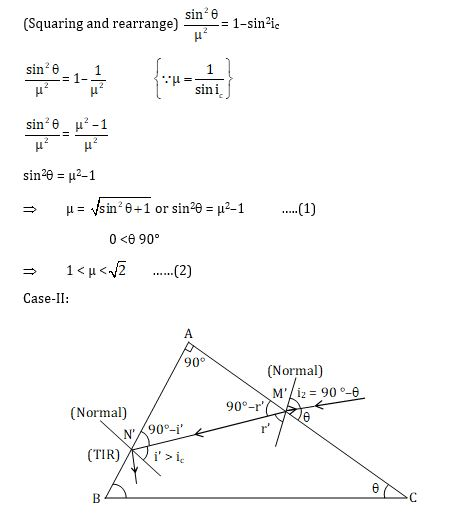In case-II from triangle AM’N’ = 90° + 90–r’ + 90–i’ = 180°

⇒ i’ + r’ = 90°

Applying Snell’s law, $\frac{sin i_{2}}{sin r'} = \mu$

$\frac{sin (90 - \theta )}{sin (90 - i')} = \mu$

$\frac{cos \theta }{\mu } = cos\: i'$ …..(a)

Now according to condition of total internal reflection,

i’ > ic

sin i’ > sin ic

sin2i’ > sin2ic

$1 - cos^{2} i' > \frac{1}{\mu ^{2}}\left \{ \mu =\frac{1}{sini_{c}} \right \}$

$1 - \frac{1}{\mu ^{2}}cos^{2}\: i'$ …..(b)

From equation (a) and (b),

$\frac{\mu ^{2} - 1}{\mu ^{2}}> \frac{cos^{2}\theta }{\mu ^{2}}$

µ2–1> cos2 θ

µ2 >cos2 θ+ 1

µ2 >1–sin2θ+ 1

µ2 >2–sin2θ

Substituting the value from equation (1),

µ2 > 2–(µ–1)

µ2 >2–µ2 + 1

2 > 3

$\mu > \sqrt{\frac{3}{2}}$ …..(3)

So, from equation (2) and (3),

$\sqrt{\frac{3}{2}} < \mu < \sqrt{2}$

So, the correct option is (a).

Question 30. A smaller cube with side b (depicted by dashed lines) is excised from a bigger uniform cube with side a as shown below such that both cubes have a common vertex P. Let X = a/b. If the center of mass of the remaining solid is at the vertex O of smaller cube then X satisfies.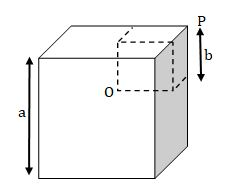1. a) X3 – X2 –X – 1 = 0
2. b) X2 –X – 1 = 0
3. c) X3 + X2 –X – 1 = 0
4. d) X3 – X2 –X + 1 = 0

Solution: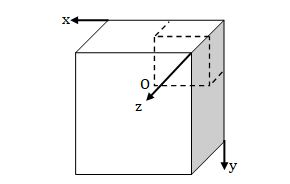∴ Centre of mass at point O is given by $\frac{m_{1}x_{1}+ m_{2}x_{2}}{m_{1}+m_{2}}$

Here, we have to find the equation in terms of X.

∵Density (ρ) = mass(m) / volume (V)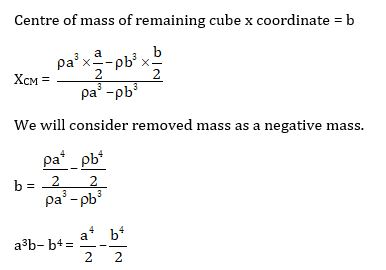2a3b–2b4 = a4–b4

Put a = bx ⇒ 2b4x3 b–2b4 = b4x4–b4

2x3 –1= x4

2x3 –2 + 1 = x4

2(x3–1) = (x2–1) (x2+1)

2(x–1)(x2+1+x) = (x–1) (x+1) (x2+1)

2x2 + 2 + 2x = x3 + x + x2+1

x3–x2–x+1=0

So, the correct option is (a).

### KVPY SX 2016 Physics Paper with Solutions